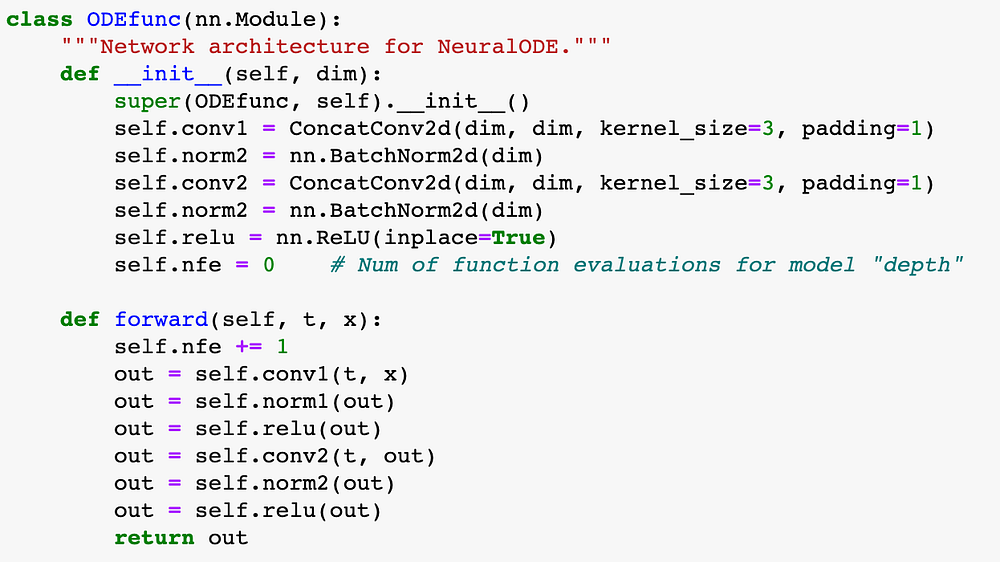# Intro to Neural ODEs: Part 3 — The Basics

Original article can be found here (source): Deep Learning on Medium# Intro to Neural ODEs: Part 3 — The Basics

## In this part, we take a look at the basics of how a Neural ODE works.

Last time, we saw how a ResNet is very similar to Euler’s method. Then, we applied this idea backwards to obtain the following differential equation.

This equation now describes the dynamics of our model. It is a neural ODE. Basically, it is the continuous analog of a ResNet.

You may be wondering — why is this important? The reason that Neural ODEs are so interesting is that they marry two seemingly disparate concepts: deep learning and differential equations. By combining the two, we can rely on hundreds of years of mathematical research within the fields of differential equations and dynamical systems.

Given an initial state (input) for the model, we can use numerical methods to approximate the solution and solve for the output — this means making predictions! One option is to use Euler’s method again. However, we can also use much more complex and more accurate diffeq solvers to obtain the best predictions possible.

A natural followup question is — how do we train it? This is a bit more complicated and might be the topic of a future blog post. For now, it is enough to know that the model is trained with the adjoint method. This involves using another numerical solver to run backwards through time (backpropagating) and updating the model’s parameters.